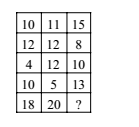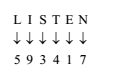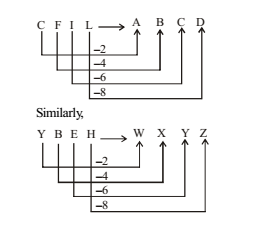# RRB ALP 2018 Practice Test Papers | Reasoning Questions (Day-37)

Dear Aspirants, Here we have given the Important RRB ALP & Technicians Exam 2018 Practice Test Papers. Candidates those who are preparing for RRB ALP 2018 can practice these Reasoning Questions to get more confidence to Crack RRB 2018 Examination.

[WpProQuiz 2119]

Click “Start Quiz” to attend these Questions and view Solutions

1. Find the odd word/letters/ number pair from the given alternatives.

(a) PORTRAIT

(b) DRAW

(c) PAINT

(d) SKETCH DIRECTIONS

1. In a line, Naresh is 17th from the left & 22nd from the right. How many students are there in the line?

(a) 40

(b) 38

(c) 39

(d) 37

1. Some equations have been solved on the basis of certain system. Find the correct answer for the unsolved equations on that basis? If 72 × 19 = 23, 13 × 48 = 35 and 16 × 43 = 18 then 39 × 22 =?

(a) 27

(b) 51

(c) 31

(d) 21

1. If LISTEN is coded as 593417 then SILENT is code as:

(a) 591734

(b) 391754

(c) 591743

(d) 395174

1. In a certain code language NATIONALISM is written as OINTANMSAIL. How is DEPARTMENTS written in that code?

1. CFIL: ABCD:: _?_ : WXYZ

(a) ZYXW

(b) DCBA

(c) YBEH

(d) XURO

1. From the given alternative words, select the word which cannot be formed using the letters of the given word:

DISINTEGRATION

(a) SIGN

(b) SIGNATURE

(c) INTERN

(d) SINGER

1. Select the missing number from the given responses.(a) 21

(b) 20

(c) 23

(d) 22

1. If 64÷14 = 5,92÷31 = 7, 26 ÷ 11 = 6, then 56÷22 = _?_

(a) 11

(b) 39

(c) 7

(d) 36

1. Select the related letter/word/ number from the given alternatives:

Zoology: Animal:: Psychology: _?_

(a) Animal

(b) Human-being

(c) Animal and human-being

(d) Plant

We used to draw, sketch or paint a portrait.

Naresh is 17th from left and 22nd from the right. So, total number of students in the line = 17 + 22 – 1 = 38

72 × 19 = 23 => (7 × 2 + 1 × 9 = 23)

13 × 48 = 35 => (1 × 3 + 4 × 8 = 35)

16 × 43 = 18 => (1 × 6 + 4 × 3 = 18)

So, 39 × 22 =? => (3 × 9 + 2 × 2 = 31)

1. Answer: (d)Divide the word into two groups of five letters each. The first five letters are in group I and the last five letters are in group II. Now, for its coding the middle letters remain unchanged. While the letters in each group change their position as 1 -> 3, 2 -> 5, 3 -> 4, 4->2 and 5->1.SIGNATURE cannot be formed as reference word doesnot have ‘U’ alphabet.

First Column-> 10 + 12 + 4 + 10 = 36

36/2= 18

Second Column ->.11 + 12 + 12 + 5 = 40

40/ 2= 20

Third Column-> 15 + 8 + 10 + 13 = 46

46 /2= 23

r = 3 cm

6 + 4 = 10; 1 + 4 = 5; 10 – 5 = 5

9 + 2 = 11; 3 + 1 = 4; 11 – 4 = 7

2 + 6 = 8; 1 + 1 = 2; 8 – 2 = 6

5 + 6 = 11; 2 + 2 = 4, 11 – 4 = 7

Zoology is the scientific study of animals. Similarly, Psychology is study of the mind and how it functions. It is the study of human and animals behaviour.

RRB ALP 2018 Practice Test Papers | Reasoning Questions (Day-1)

RRB ALP 2018 Practice Test Papers | Reasoning Questions (Day-2)

RRB ALP 2018 Practice Test Papers | Reasoning Questions (Day-3)

RRB ALP 2018 Practice Test Papers | Reasoning Questions (Day-4)

RRB ALP 2018 Practice Test Papers | Reasoning Questions (Day-5)

RRB ALP 2018 Practice Test Papers | Reasoning Questions (Day-6)

RRB ALP 2018 Practice Test Papers | Reasoning Questions (Day-7)

RRB ALP 2018 Practice Test Papers | Reasoning Questions (Day-8)

RRB ALP 2018 Practice Test Papers | Reasoning Questions (Day-9)

RRB ALP 2018 Practice Test Papers | Reasoning Questions (Day-10)

RRB ALP 2018 Practice Test Papers | Reasoning Questions (Day-11)

RRB ALP 2018 Practice Test Papers | Reasoning Questions (Day-12)

RRB ALP 2018 Practice Test Papers | Reasoning Questions (Day-13)

RRB ALP 2018 Practice Test Papers | Reasoning Questions (Day-14)

RRB ALP 2018 Practice Test Papers | Reasoning Questions (Day-15)

RRB ALP 2018 Practice Test Papers | Reasoning Questions (Day-16)

RRB ALP 2018 Practice Test Papers | Reasoning Questions (Day-17)

RRB ALP 2018 Practice Test Papers | Reasoning Questions (Day-18)

RRB ALP 2018 Practice Test Papers | Reasoning Questions (Day-19)

RRB ALP 2018 Practice Test Papers | Reasoning Questions (Day-20)

RRB ALP 2018 Practice Test Papers | Reasoning Questions (Day-21)

RRB ALP 2018 Practice Test Papers | Reasoning Questions (Day-22)

RRB ALP 2018 Practice Test Papers | Reasoning Questions (Day-23)

RRB ALP 2018 Practice Test Papers | Reasoning Questions (Day-24)

RRB ALP 2018 Practice Test Papers | Reasoning Questions (Day-25)

RRB ALP 2018 Practice Test Papers | Reasoning Questions (Day-26)

RRB ALP 2018 Practice Test Papers | Reasoning Questions (Day-27)

RRB ALP 2018 Practice Test Papers | Reasoning Questions (Day-28)

RRB ALP 2018 Practice Test Papers | Reasoning Questions (Day-29)

RRB ALP 2018 Practice Test Papers | Reasoning Questions (Day-30)

RRB ALP 2018 Practice Test Papers | Reasoning Questions (Day-31)

RRB ALP 2018 Practice Test Papers | Reasoning Questions (Day-32)

RRB ALP 2018 Practice Test Papers | Reasoning Questions (Day-33)

RRB ALP 2018 Practice Test Papers | Reasoning Questions (Day-34)

RRB ALP 2018 Practice Test Papers | Reasoning Questions (Day-35)

RRB ALP 2018 Practice Test Papers | Reasoning Questions (Day-36)